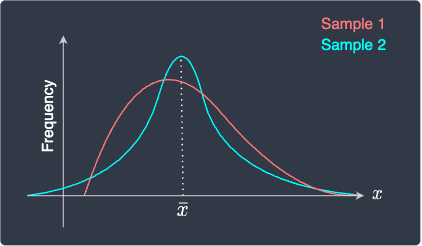search
Search
Unlock 100+ guides
search toc
close
account_circle
Profile
exit_to_app
Sign out
search
keyboard_voice
close
Searching Tips
Search for a recipe:
"Creating a table in MySQL"
Search for an API documentation: "@append"
Search for code: "!dataframe"
Apply a tag filter: "#python"
Useful Shortcuts
/ to open search panel
Esc to close search panel
to navigate between search results
d to clear all current filters
Enter to expand content previewDoc SearchCode Search BetaSORRY NOTHING FOUND!
mic
Start speaking...Voice search is only supported in Safari and Chrome.
Shrink
Navigate to

# Comprehensive Guide on Sample Mean

schedule Aug 11, 2023
Last updated
local_offer
Probability and Statistics
Tags
mode_heat
Master the mathematics behind data science with 100+ top-tier guides
Start your free 7-days trial now!

To estimate the population mean, we can collect some observations from the population to form a sample. We would expect the average of these observations, which is called the sample mean, to be a good estimate of the true population mean.

Theorem.

# Sample mean

The mean of a sample of observations $(x_1,x_2,...,x_n)$ is computed by:

$$\bar{x}=\frac{1}{n} \sum^n_{i=1}x_i$$

$\bar{x}$ is known as the sample mean.

Example.

## Computing the sample mean

Compute the sample mean of the following sample:

$$(2,4,8,2)$$

Solution. The size of the sample is $n=4$. Using the formula for the sample mean:

\begin{align*} \bar{x}&=\frac{1}{4}\sum^4_{i=1}x_i\\ &=\frac{1}{4}(2+4+8+2)\\ &=4 \end{align*}

Therefore, the mean of our sample is $4$.

# Samples with different distribution can have the sample mean

The sample mean is a measure of the central tendency of a sequence of values. However, the sample mean itself is not sufficient to describe the distribution of the values since vastly different distributions can have the same sample mean. For instance, consider the following frequency distribution of two samples:Here, their distributions look different but they have the same sample mean. Therefore, when numerically describing a sample, we should additionally quote other statistical measures such as the sample variance.

# Properties of the sample mean

Definition.

## Expected value of the sample mean

The expected value of the sample mean $\bar{X}$ is equal to the population mean $\mu$, that is:

$$\mathbb{E}(\bar{X})=\mu$$

Equivalently, we say that the expected value is an unbiased estimator of the population mean.

Proof. From the definition of sample mean, we have that:

$$\bar{X}=\frac{1}{n} \sum^n_{i=1}x_i$$

Taking the expected value of both sides and using the properties of expected values to simplify:

\begin{align*} \mathbb{E}(\bar{X})&= \mathbb{E}\Big(\frac{1}{n} \sum^n_{i=1}X_i\Big)\\ &=\frac{1}{n} \sum^n_{i=1}\mathbb{E}(X_i) \end{align*}

Since the expected value of $X_i$ is the population mean $\mu$, we have that:

\begin{align*} \mathbb{E}(\bar{X})&= \frac{1}{n} \sum^n_{i=1}\mu\\ &= \frac{1}{n} \cdot{n\mu}\\ &=\mu \end{align*}

This completes the proof.

Theorem.

## Variance of the sample mean

The variance of the sample mean is:

$$\mathbb{V}(\bar{X})=\frac{\sigma^2}{n}$$

Where:

• $\sigma^2$ is the population variance.

• $n$ is the sample size.

Proof. The variance of the sample mean is:

\begin{align*} \mathbb{V}(\bar{X}) &=\mathbb{V}\Big(\frac{1}{n}\sum^{n}_{i=1}X_i\Big)\\ &=\frac{1}{n^2}\cdot\mathbb{V}\Big(\sum^{n}_{i=1}X_i\Big)\\ \end{align*}

Because $X_1,X_2,\cdots,X_n$ are independent, we know from theoremlink that we can swap the position of the summation and variance to get:

\begin{align*} \mathbb{V}(\bar{X})&=\frac{1}{n^2}\sum^{n}_{i=1}\mathbb{V}(X_i)\\ &=\frac{1}{n^2}\sum^{n}_{i=1}\sigma^2\\ &=\frac{1}{n^2}(n\sigma^2)\\ &=\frac{\sigma^2}{n}\ \end{align*}

This completes the proof.

Theorem.

## Standard error of the sample mean

The standard error of the sample mean, which is defined as the standard deviation of the sample mean, is given by:

$$\text{SE}(\bar{X})= \sqrt{\mathbb{V}(\bar{X})} =\frac{\sigma}{\sqrt{n}}$$

Where:

• $\sigma$ is the population standard deviation.

• $n$ is the sample size.

Proof. We already know that the variance of the sample mean is:

$$\mathbb{V}(\bar{X})=\frac{\sigma^2}{n}$$

To obtain the standard error, we take the square root of the variance:

$$\text{SE}(\bar{X})=\frac{\sigma}{\sqrt{n}}$$

This completes the proof.

# Computing the sample mean in Python

Computing the sample mean is easy using Python's numpy library. Import the library, and use the mean(~) method:

 import numpy as npnp.mean([2,4,8,2]) 4.0 
thumb_up
thumb_down
Comment
Citation
Ask a question or leave a feedback...
thumb_up
0
thumb_down
0
chat_bubble_outline
0
settings
Enjoy our search
Hit / to insta-search docs and recipes!